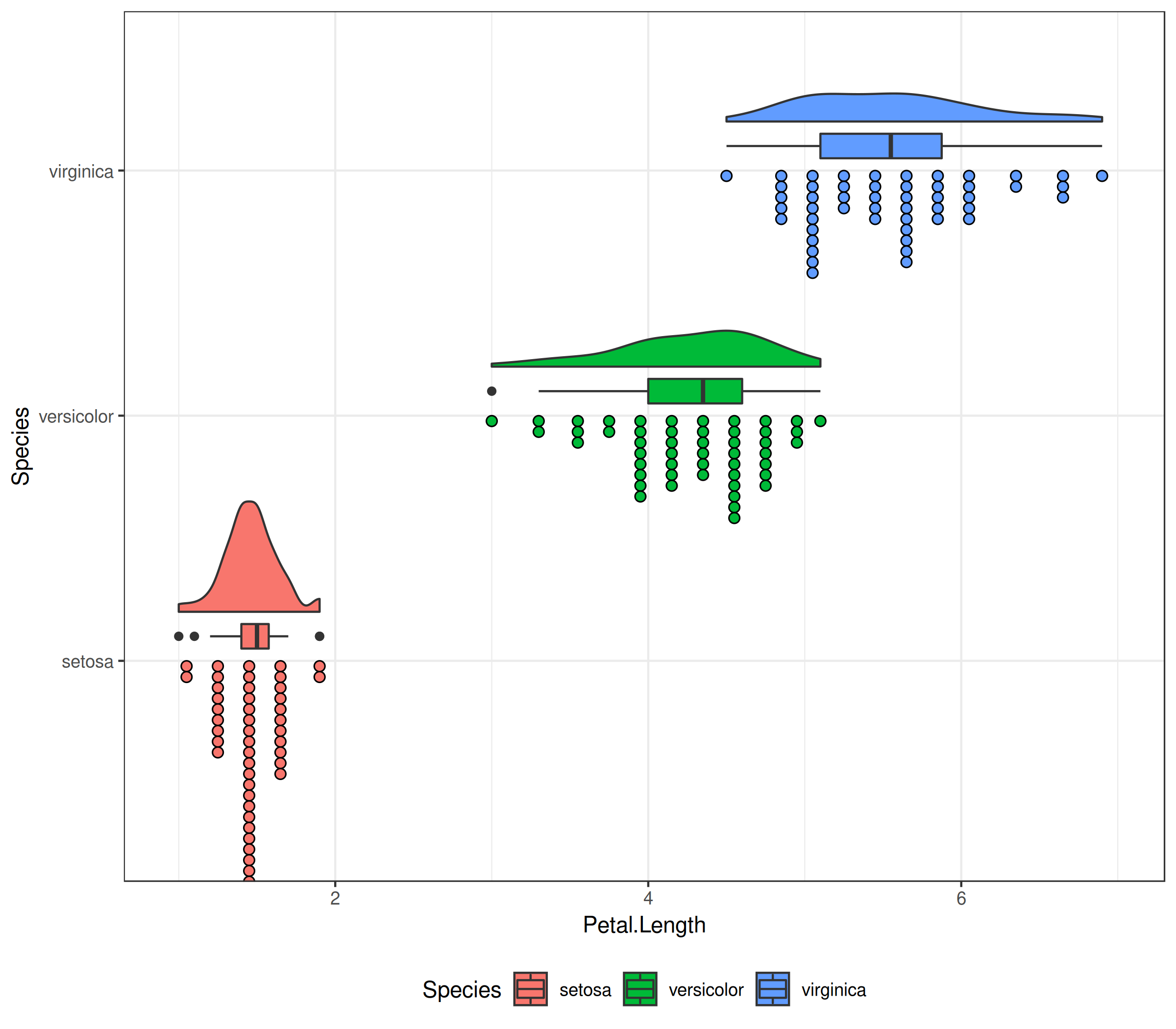• boxplot + raw data
• violin plot + boxplot
• violin plot + raw data
• violin plot + boxplot + raw data

ggridges：一种波涛汹涌，哦不对，是山峰叠峦的可视化方式》本身长得就像半个violin，这个包现在也支持raincloud，不过我还是喜欢单一的元素然后自己拼。

### violin + boxplot + raw data

``````require(ggplot2)
ggplot(iris, aes(Species, Petal.Length, fill=Species))  +
geom_violin(alpha=.5) +
geom_boxplot(width=.1) +
geom_jitter()
``````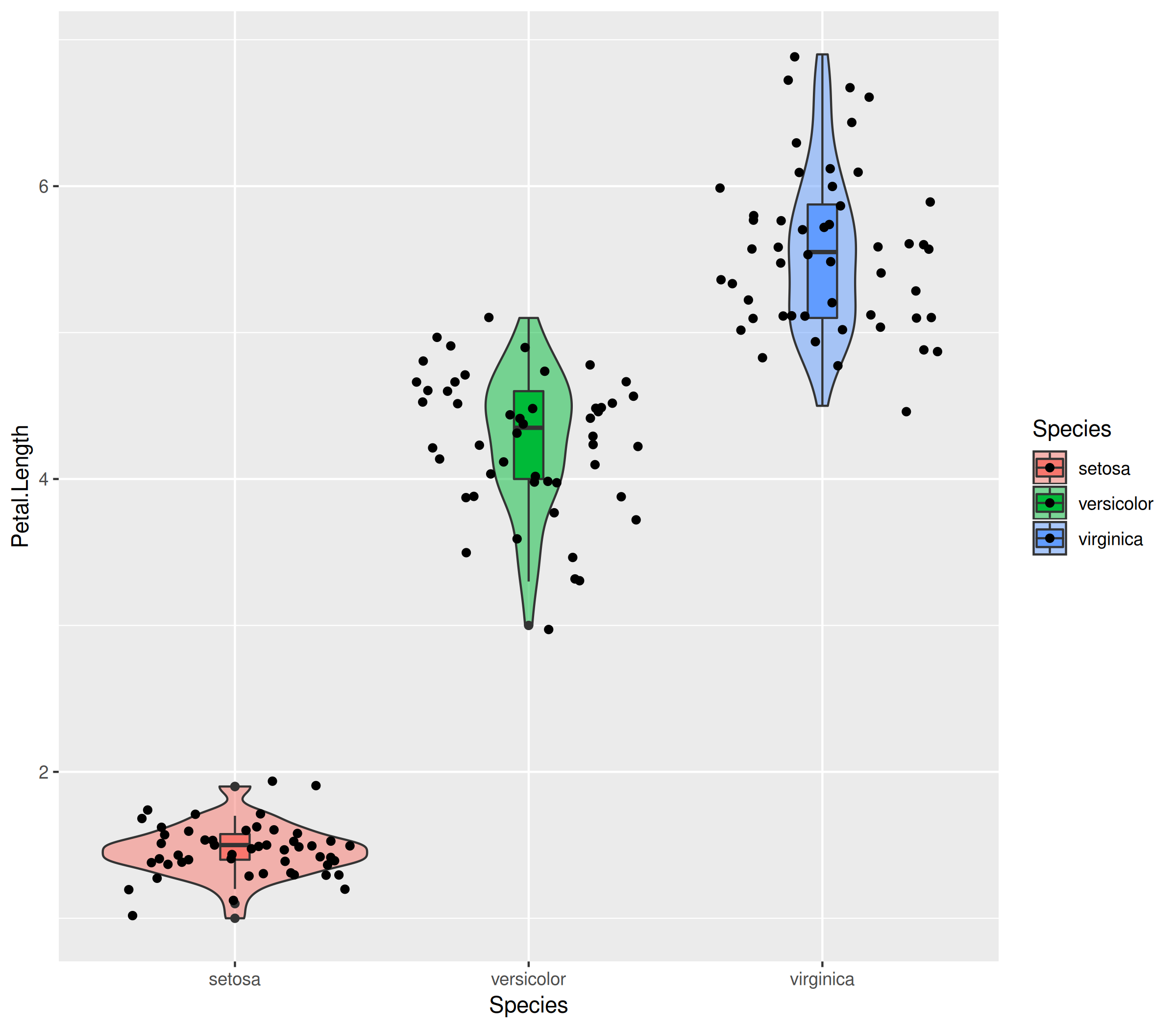### violin + mean+-sd + raw data

``````require(dplyr)
d <- group_by(iris, Species) %>%
summarize(mean = mean(Petal.Length),
sd = sd(Petal.Length))

ggplot(iris, aes(Species, Petal.Length, fill=Species))  +
geom_violin(alpha=.5) +
geom_jitter() +
geom_pointrange(aes(y=mean, ymin=mean-sd, ymax=mean+sd, color=Species), data=d, size=2)
``````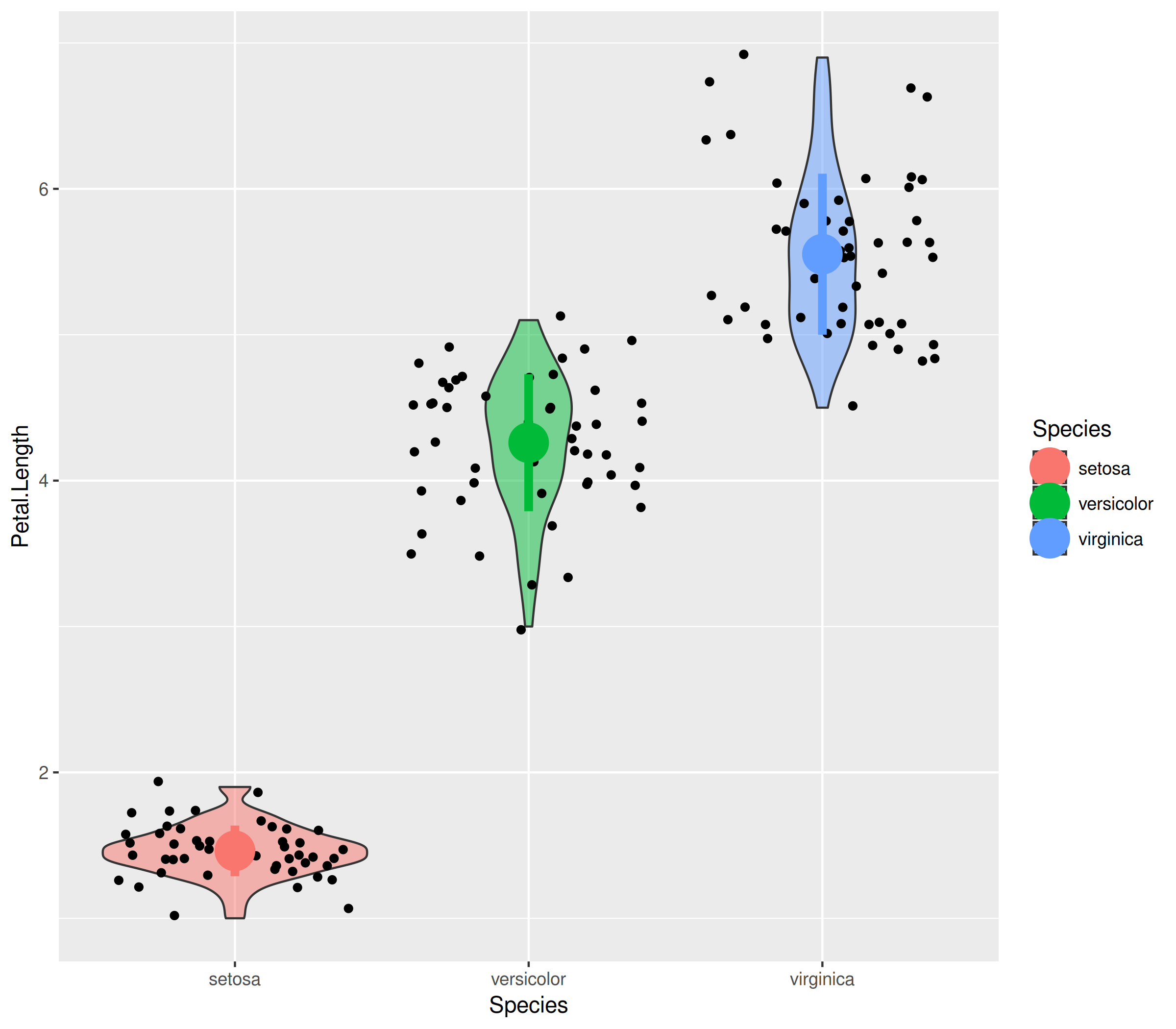### 云雨图1：加上均值和标准误差。

``````## devtools::install_github("GuangchuangYu/gglayer")
require(gglayer)

ggplot(iris, aes(Species, Petal.Length, fill=Species))  +
geom_flat_violin(position=position_nudge(x=.2)) +
geom_jitter(aes(color=Species), width=.15) +
geom_pointrange(aes(y=mean, ymin=mean-sd, ymax=mean+sd),
data=d, size=1, position=position_nudge(x=.25)) +
coord_flip() + theme_bw() +
theme(legend.position="bottom")
``````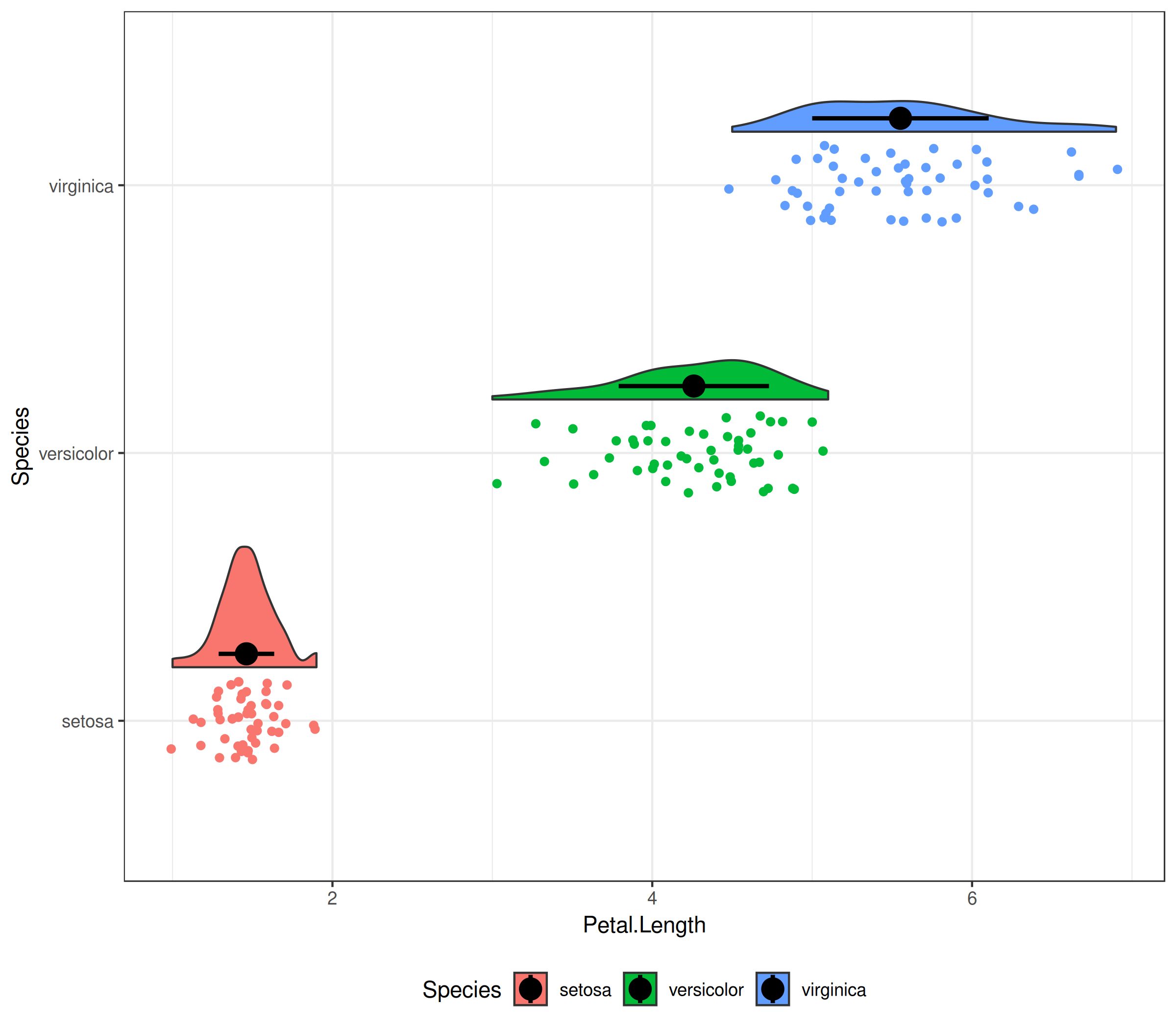### 云雨图2：加上boxplot

``````ggplot(iris, aes(Species, Petal.Length, fill=Species))  +
geom_flat_violin(position=position_nudge(x=.3)) +
geom_jitter(aes(color=Species), width=.15) +
geom_boxplot(width=.1, position=position_nudge(x=.22)) +
coord_flip() + theme_bw() +
theme(legend.position="bottom")
``````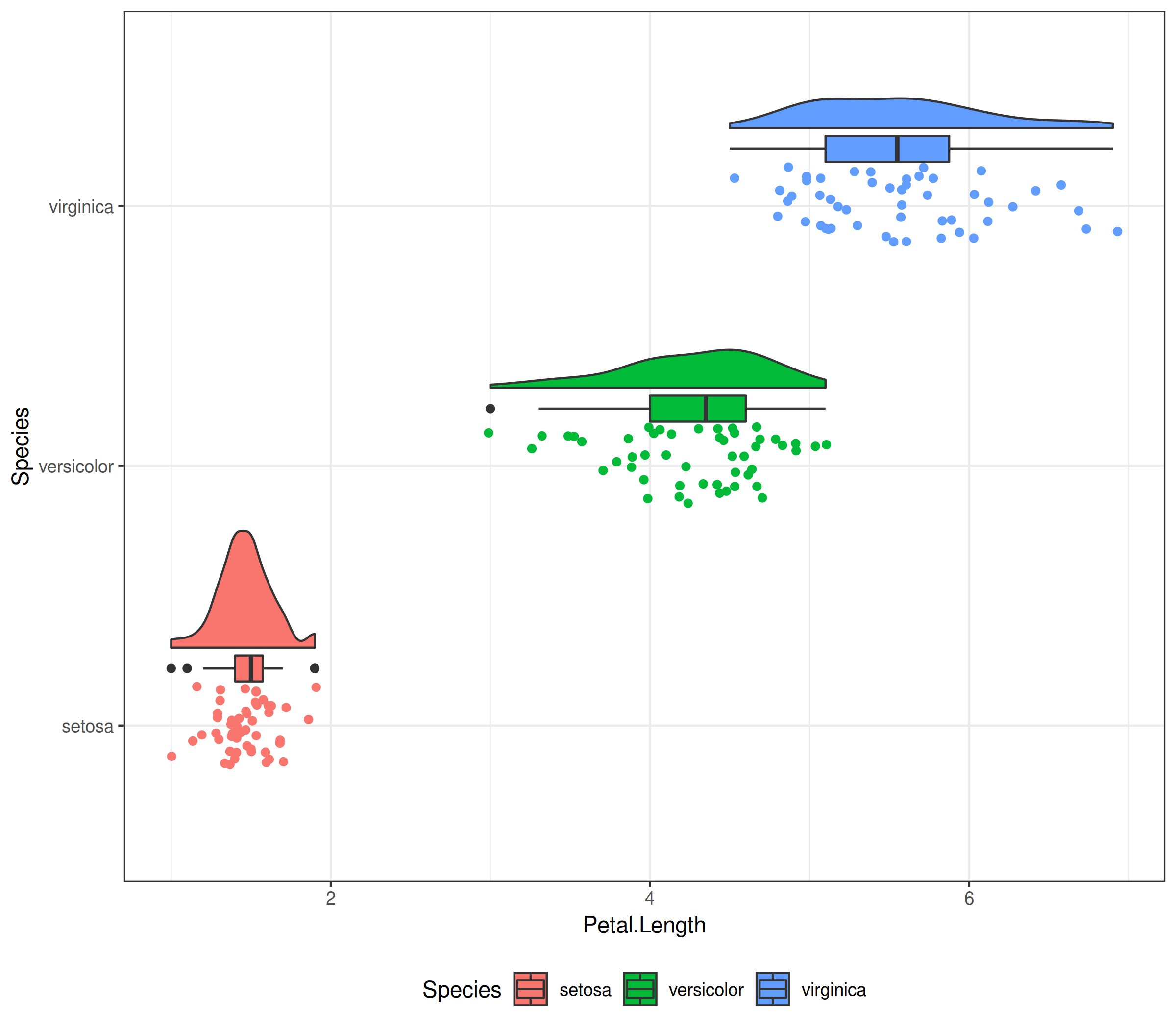### 云雨图3：用堆叠的点图当雨点

``````ggplot(iris, aes(Species, Petal.Length, fill=Species))  +
geom_flat_violin(position=position_nudge(x=.2)) +
geom_dotplot(binaxis="y", stackdir="down", dotsize=.35) +
geom_boxplot(width=.1, position=position_nudge(x=.1)) +
coord_flip() + theme_bw() +
theme(legend.position="bottom")
``````